# AIIMS Full Mock Test 18

## 200 Questions MCQ Test AIIMS Mock Tests & Previous Year Papers | AIIMS Full Mock Test 18

Description
This mock test of AIIMS Full Mock Test 18 for NEET helps you for every NEET entrance exam. This contains 200 Multiple Choice Questions for NEET AIIMS Full Mock Test 18 (mcq) to study with solutions a complete question bank. The solved questions answers in this AIIMS Full Mock Test 18 quiz give you a good mix of easy questions and tough questions. NEET students definitely take this AIIMS Full Mock Test 18 exercise for a better result in the exam. You can find other AIIMS Full Mock Test 18 extra questions, long questions & short questions for NEET on EduRev as well by searching above.
QUESTION: 1

Solution:
QUESTION: 2

Solution:
QUESTION: 3

### In a given circuit as shown the two input waveform A and B are applied simultaneously. The resultant waveform Y is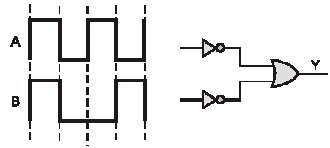Solution:
QUESTION: 4

In circuit in following fig. the value of Y is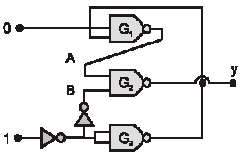Solution:
QUESTION: 5

In the following circuit, a voltmeter V is connected across a lamp L. What change would occur in voltmeter reading if the resistance R is reduced in value.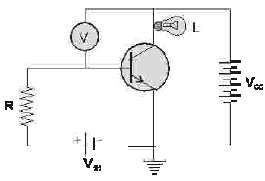Solution:
QUESTION: 6

In the study of transistor as an amplifier, if a = Ic / Ie and b = Ic / Ib, where Ic ,Ib and Ie are the collector, base and emitter currents, then

Solution:
QUESTION: 7

For the given combination of gates, if the logic state of inputs A, B, C are as follows A = B = C = 0 and A = B = 1, C = 0 then the logic states o output D are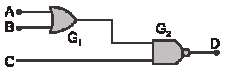Solution:
QUESTION: 8

Which logic gate is represented by the following combination of logic gates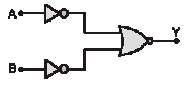Solution:
QUESTION: 9

In the diagram, the input is across the terminals A and C and the output is across the terminals B and D, then the output isSolution:
QUESTION: 10

The phase difference between input and output voltages of a CE circuit is

Solution:
QUESTION: 11

Which of the following is true

Solution:
QUESTION: 12

The energy band diagrams for three semiconductor samples of silicon are as shown. We can then assert that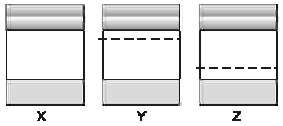Solution:
QUESTION: 13

Which of the following statements is not true

Solution:
QUESTION: 14

The dominant mechanisms for motion of charge carriers in forward and reverse biased silicon P-N junctions are

Solution:
QUESTION: 15

Which one is reverse-biased

Solution:
QUESTION: 16

Consider the following statements A and B and identify the correct choice of the given answers
A: The width of the depletion layer in a P-N junction diode increases in forwards bias
B : In an intrinsic semiconductor the fermi energy level is exactly in the middle of the forbidden gap

Solution:
QUESTION: 17

Which of the following ray diagram show physically possible refraction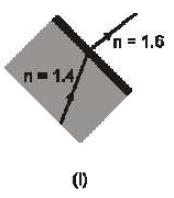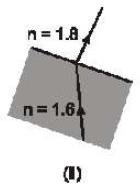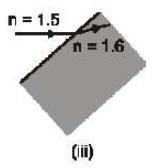Solution:
QUESTION: 18

When the rectangular metal tank is filled to the top with an unknown liquid, as observer with eyes level with eyes level with the top of the tank can just see the corner E; a ray that refracts towards the observer at the top surface of the liquid is shown. The refractive index of the liquid will be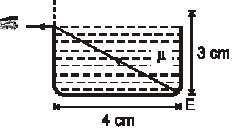Solution:
QUESTION: 19

PQR is a right angled prism with other angles as 60º a d 30º. Ref ractive index of prism is 1.5 PQ has a thin layer of liquid. Light falls normall on the face PR. For total internal reflection, maximum refractive index of liquid is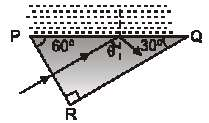Solution:
QUESTION: 20

When a ray is refracted from one medium to another, th wavelength changes from 6000 Å to 4000 Å. The critical angle for the interface will be

Solution:
QUESTION: 21

Two thin lenses, when in contact, produce a combination of power + 10 D. When they are 0.25 m apart, the power reduces to + 6D. The focal lengths of the lenses (in m) are

Solution:
QUESTION: 22

The plane faces of two identical plane convex lances, each with focal length f are pressed against each other using an optical glue to forma usual convex lens. The distance from the optical center at which an object must be placed to obtain the image same as the size if object is

Solution:
QUESTION: 23

A parallel beamof light emerges fromthe opposite surface of the sphere when a point source of light lies at the surface of the sphere. The refractive index of the sphere is

Solution:
QUESTION: 24

A thin rod of 5 cmlength is kept along the axis of a concave mirror of 10 cm focal length such that its image is real and magnified and one end touches the rod. Its magnification will be

Solution:
QUESTION: 25

A lens when placed on a plane mirror then object needle and its image coincide at 15 cm. The focal length of the lens is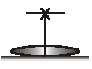Solution:
QUESTION: 26

Flint glass prism is joined by a crown glass prism to produce dispersion without deviation. The refractive indices of these for mean rays are 1.602 and 1.500 respectively. Angle of prism of flint prism is 100, then the angle of prism for crown prism will be

Solution:
QUESTION: 27

An astronomical telescope has an angular magnification of magnitude 5 for distant objects. The separation between the objective and the eye-piece is 36 cm and the final image is formed at infinity. The focal length f0 of the objective and fe of the eye-piece are

Solution:
QUESTION: 28

The magnifying power of compound microscope in terms of the magnification m0 due to objective and magnifying power mE by the eye-piece is given by

Solution:
QUESTION: 29

With a simple microscope, if the final image is located at the least distance of distinct vision (i.e., D) from the eye placed close to the lens, then the magnifying power is

Solution:
QUESTION: 30

If in a Young's double slit experiment, the slit distance is 3 cm, the separation between slits and screen is 70cm and wavelength of light is 1000 Å , then fringe width will be

Solution:
QUESTION: 31

If a mica sheet of thickness t and refractive index is placed in the path of one of interfering beam in a double slit experiment then displacement of fringes will be :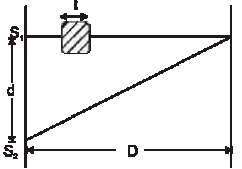Solution:
QUESTION: 32

Two periodic waves of intensities I1 and I2 pass through a region at the same time in the same direction. The sum of the maximum and minimum intensities is

Solution:
QUESTION: 33

If the frequency of light in a photoelectric effect is doubled, then stopping potential will

Solution:
QUESTION: 34

An α-particle moves along a circular path of radius 0.83 cm in a magnetic field of 0.25 Wb/m2. The de-Broglie wavelength associated with it will be

Solution:
QUESTION: 35

If the frequency of K line of the element having Z = 64 is (vα), and the frequency of Kα line of the element having Z = 80 is vα , then the ratio of their frequencies will be about

Solution:
QUESTION: 36

If the mass of proton = 1.008 a.m.u. and mass of neutron = 1.009 a.m.u. then binding energy per nucleon for 4Be9 (mass = 9.012 amu) would be-

Solution:
QUESTION: 37

The binding energies of two nuclei Pn and Q2n and x and y joules. If 2x > y then the energy released in the reaction Pn + Pn → Q2n, will be

Solution:
QUESTION: 38

1014 fissions per second are taking place in a nuclear reaction having efficiency 40%. The energy released per fission is 250 MeV. The power output of the reactor is

Solution:
QUESTION: 39

The count rate of 10g of radioactive material was measured at different times and this has been shown in fig. The half life of material and the total counts (approximately) in the first half life period, respectively are-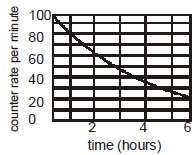Solution:
QUESTION: 40

A radioactive element ThA (84Po216) can undergo α and β are type of disintegrations with half-lives, T1 and T2 respectively. Then the half-life of ThA is

Solution:
QUESTION: 41

The following questions consist of two statements one  labelled ASSERTION (A) and the another labelled REASON (R). Select the correct answers to these questions from the codes given below :

Assertion: Work function of aluminium is 4.2 eV. Emiss on of electrons will not be possible if two photons each of energy 2.5 eV collide with free electrons of aluminium.
Reason : For photoelectric emission the energy of each photon should be greater than or equal to the work function of aluminium

Solution:
QUESTION: 42

The following questions consist of two statements one  labelled ASSERTION (A) and the another labelled REASON (R). Select the correct answers to these questions from the codes given below :

Assertion: Kinetic energy of photo electrons emitted by a photosensitive surface depends upon the intensity of incident photon.
Reason : The ejection of electrons from metallic surface is possible with frequency of incident photon below the threshold frequency.

Solution:
QUESTION: 43

The following questions consist of two statements one  labelled ASSERTION (A) and the another labelled REASON (R). Select the correct answers to these questions from the codes given below :

Assertion : Photosensitivity of a metal is high if its work function is small.
Reason : Work function = hf0 where f0 is the threshold frequency

Solution:
QUESTION: 44

The following questions consist of two statements one  labelled ASSERTION (A) and the another labelled REASON (R). Select the correct answers to these questions from the codes given below :

Assertion : Average power consumed in an ac circuit is equal to average power consumed by resistors in the circuit.
Reason : Average power consumed by capacitor and inductor is zero

Solution:
QUESTION: 45

The following questions consist of two statements one  labelled ASSERTION (A) and the another labelled REASON (R). Select the correct answers to these questions from the codes given below :

Assertion : The electrostatic energy stored in capacitor plus magnetic energy stored in inductor will always be zero in a series LCR circuit driven by ac voltage source under condition of resonance.
Reason : The complete voltage of ac source appears eqully across the all the elements in a series LCR circuit driven by ac voltage source under condition of resonance.

Solution:
QUESTION: 46

The following questions consist of two statements one  labelled ASSERTION (A) and the another labelled REASON (R). Select the correct answers to these questions from the codes given below :

Assertion : Two coaxial conducting rings of different radii are placed concentric in space. The mutual inductance of both the rings is maximum if the rings are also coplanar.
Reason : For two coaxial conducting rings of different radii, the magnitude of magnetic flux in one ring due to current in other ring is maximum when both rings are coplanar

Solution:
QUESTION: 47

The following questions consist of two statements one  labelled ASSERTION (A) and the another labelled REASON (R). Select the correct answers to these questions from the codes given below :

Assertion : Consider the arrangement shown below. A smooth conducting rod, CD, is lying on a smooth U-shaped conducting wire making good electrical contact with it. The U-shape conducting wire is fixed and lies in horizontal plane. There is a uniformand constant magnetic field B in vertical direction (perpendicular to plane of page in figure). If the magnetic field strength is decreased, the rod moves towards right.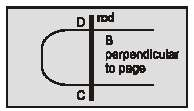Reason : In the situation of Assertion, the direction in which the rod will slide is that which tends to maintain constant flux through the loop. Providing a larger loop area counteracts the decrease in magnetic flux. So the rod moves to the right independent of the fact that the direction of magnetic field is into the page or out of the page

Solution:
QUESTION: 48

The following questions consist of two statements one  labelled ASSERTION (A) and the another labelled REASON (R). Select the correct answers to these questions from the codes given below :

Assertion : A dip circle (used for dip measurement) is taken to geomegnetic equator. The needle is allowed to move in a vertical plane The needle will stay in any direction when it is relesed
Reason : At geomagnetic equator Bv = zero

Solution:
QUESTION: 49

The following questions consist of two statements one  labelled ASSERTION (A) and the another labelled REASON (R). Select the correct answers to these questions from the codes given below :

Assertion : The logic gate NOT can be built using diode.
Reason : The output voltage and the input voltage of the diode have 180º phase difference.

Solution:
QUESTION: 50

The following questions consist of two statements one  labelled ASSERTION (A) and the another labelled REASON (R). Select the correct answers to these questions from the codes given below :

Assertion : The number of electrons in a P-type silicon semiconductor is less than the number of electron in a pure silicon semiconductor at room temperature.
Reason : It is due to law of mass action.

Solution:
QUESTION: 51

The following questions consist of two statements one  labelled ASSERTION (A) and the another labelled REASON (R). Select the correct answers to these questions from the codes given below :

Assertion : In a common emitter transistor amplifier the input current is much less than the output current.
Reason : The common emitter transistor amplifier has very high input impedance.

Solution:
QUESTION: 52

The following questions consist of two statements one  labelled ASSERTION (A) and the another labelled REASON (R). Select the correct answers to these questions from the codes given below :

Assertion : If the temperature of a semiconductor is increased then it's resistance decreases.
Reason : The energy gap between conduction band and valence band is very small.

Solution:
QUESTION: 53

The following questions consist of two statements one  labelled ASSERTION (A) and the another labelled REASON (R). Select the correct answers to these questions from the codes given below :

Assertion : The temperature coefficient of resistance is positive for metals and negative for P-type semiconductor.
Reason : The effective charge car riers in metals are n gatively charged whereas in Ptype semiconductor they are positively charged.

Solution:
QUESTION: 54

The following questions consist of two statements one  labelled ASSERTION (A) and the another labelled REASON (R). Select the correct answers to these questions from the codes given below :

Assertion : Light emitting diode (LED) emits spontaneous radiation.
Reason : LED are forward biased p-n junctions.

Solution:
QUESTION: 55

The following questions consist of two statements one  labelled ASSERTION (A) and the another labelled REASON (R). Select the correct answers to these questions from the codes given below :

Assertion : The energy gap between the valence band and conduction band is greater in silicon than in germanium.
Reason : Thermal energy produces fewer minority carriers in silicon than in germanium.

Solution:
QUESTION: 56

The following questions consist of two statements one  labelled ASSERTION (A) and the another labelled REASON (R). Select the correct answers to these questions from the codes given below :

Assertion :A p-n junction with reverse bias can be used as a photo-diode to measure light intensity.
Reason : In a reverse bias condition the current is small but it is more sensitive to changes in incident light intensity.

Solution:
QUESTION: 57

The following questions consist of two statements one  labelled ASSERTION (A) and the another labelled REASON (R). Select the correct answers to these questions from the codes given below :

Assertion : NAND or NOR gates are called digitital building blocks.
Reason : The repeated use of NAND (or NOR) gates can produce all the basic or complicated gates

Solution:
QUESTION: 58

The following questions consist of two statements one  labelled ASSERTION (A) and the another labelled REASON (R). Select the correct answers to these questions from the codes given below :

Assertion :An N-type semiconductor has a large number of electrons but still it is electrically neutral.
Reason : An N-type semiconductor is obtained by doping an intrinsic semiconductor with a pentavalent impurity.

Solution:
QUESTION: 59

The following questions consist of two statements one  labelled ASSERTION (A) and the another labelled REASON (R). Select the correct answers to these questions from the codes given below :

Assertion :No interference pattern is detected when two coherent sources are infinitely close to each other.
Reason :The fringe width is inversely proportional to the distance between the two slits.

Solution:
QUESTION: 60

The following questions consist of two statements one  labelled ASSERTION (A) and the another labelled REASON (R). Select the correct answers to these questions from the codes given below :

Assertion : Interference pattern is made by using blue light instead of red light, the fringes becomes narrower.
Reason : In Young's double slit experiment, fringe width is given by relation B =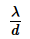Solution:
QUESTION: 61

Azero-order reaction, A → Product, with an initial concentration [A]0 has a half-life of 0.2 sec. If one starts with the concentration 2[A]0, then the half-life is :

Solution:
QUESTION: 62

The oxidation state of cobalt in the following molecule is :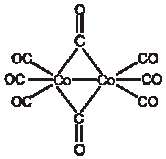Solution:
QUESTION: 63

For a tetrahedral complex [MCl4]2-, the spin-only magnetic moment is 3.83 BM. The element M is :

Solution:
QUESTION: 64

Lithium forms body centred cubic structure. Atomic radius of the lithium is 152 pm, then length of the side of its unit cell is :

Solution:
QUESTION: 65

The following data are obtained for a reaction, X + Y → Products.
Expt.        [X0]/mol  [Y0]/mol  rate/mol L-1 s–-1
1              0.25       0.25         1.0 × 10-6
2              0.50       0.25         4.0 × 10-6
3              0.25       0.50         8.0 × 10-6
The overall order of the reaction is :

Solution:
QUESTION: 66

A certain zero order reaction has k = 0.025 M s-1 for the disappearance of A. What will be the concentration of A after 15 seconds if the initial concentration is 0.50 M ?

Solution:
QUESTION: 67

Graph between log k and 1/T (k is rate constant in s-1 and T is the temperature in K) is a straight line. As shown in figure if OX = 5 and slope of the line = – 1/2.303 then Ea is :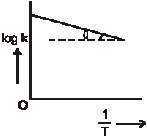Solution:
QUESTION: 68

Equivalent conductance of 1M CH3COOH is 10 ohm-1 cm2 equiv-1 and that at infinite dilution is 200 ohm-1 cm2, equiv-1. Hence % ionisation of CH3COOH is :

Solution:
QUESTION: 69

How many gm of silver will be displaced from a solution of AgNO3 by 4 gm of magnesium?

Solution:
QUESTION: 70

Diethylenetriamine is:

Solution:
QUESTION: 71

Consider the following statements and select the correct option using the codes given.
(i) [Cr(NH3)6][Cr(CN)6] and [Cr(NH3)4(CN)2] [Cr(NH3)2(CN)4] are coordination isomers.
(ii) [Cr(py)2(H2O)2Cl2]Cl and [Cr(py)2(H2O)Cl3]H2O are ligand isomers.
(iii) [Pt(NH3)4Br2]Cl2 and [Pt(NH3)4Cl2]Br2 are linkage isomers.
(iv) [NiCl2(PPh3)2] (tetrahedral) exhibits geometrical isomerism.

Solution:
QUESTION: 72

Match Column-I with Column-II and select the correct answer with respect to hybridisation using the codes given below :
Column - I                  Column - II
(Complex)                  (Hybridisation)
(I) [Au F4]-                  (p) dsp2 hybridisation
(II) [Cu(CN)4]3-           (q) sp3 hybridisation
(III) [Co(C2O4)3]3-       (r) sp3d2 hybridisation
(IV) [Fe(H2O)5NO]2+  (s) d2sp3 hybridisation

Solution:
QUESTION: 73

[Fe(en)2(H2O)2]2+ + en → complex(X). The correct statement about the complex is :

Solution:
QUESTION: 74

The solution in which the light is scattered by the particles is :

Solution:
QUESTION: 75

An arsenious sulphide sol carries a negative charge. The maximum precipitating power of this sol is possessed by :

Solution:
QUESTION: 76

Milk is an example of :

Solution:
QUESTION: 77

The stability of lyophilic colloid is due to which of the following :

Solution:
QUESTION: 78

A metal when left exposed to the atmosphere for some time becomes coated with green basic carbonate. The metal in question is

Solution:
QUESTION: 79

Which metal is present in brass, bronze and German silver

Solution:
QUESTION: 80

The two isomer X and Y with the formular Cr(H2O)5ClBr2 were taken for experiment on depression in freezing point. It was found that one mole of X gav depression corresponding to 2 moles of particles and one mole of gave depression due to 3 moles of particles. The structural formulae of X and Y respectively are :

Solution:
QUESTION: 81

Which of the following will have lowest vapour pressure :

Solution:
QUESTION: 82

In face centred cubic unit cell edge length is :

Solution:
QUESTION: 83

The order of a reaction with rate =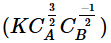equals is

Solution:
QUESTION: 84

The order of the reaction occurring by following mechanism should be
(i) A2 → A+ A (Fast)
(ii) A+ B2 → AB + B (Slow)
(iii) A + B (Fast)

Solution:
QUESTION: 85

If hydrogen electrode dipped in 2 solution of pH = 3 and pH = 6 and salt bridge is connected the e.m.f. of resulting cell is :

Solution:
QUESTION: 86

What is the correct Nernst equation for reaction taking place in the following cell :
Mg(s)| Mg2+(aq) || Cl-(aq)| Cl2(g) (1atm) /Pt

Solution:
QUESTION: 87

On mixing 3 g of non-volatile solute in 200 mL of water its boiling point (100ºC) becomes 100.52ºC. If kb for water is 0.6 K.kg/mole then molecular weight of solute is :

Solution:
QUESTION: 88

The treatment of benzene with isobutene in the presence of sulphuric acid gives :

Solution:
QUESTION: 89

The reaction of primary amine with chloroform and ethanolic solution of KOH is called :

Solution:
QUESTION: 90

The final product C, obtained in this reaction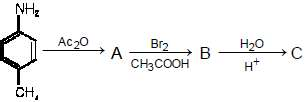would be :

Solution:
QUESTION: 91

The major product of the given reaction is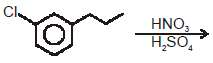Major product

Solution:
QUESTION: 92

Baeyer’s reagent is :

Solution:
QUESTION: 93

Which can give white precipitate with AgNO3

Solution:
QUESTION: 94

The major product of the given reaction is :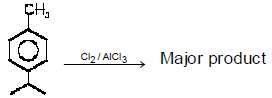Solution:
QUESTION: 95

The product (P) of the following reaction is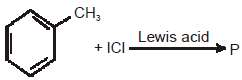Solution:
QUESTION: 96

Fluorobenzene (C6H5F) can be synthesized in the laboratory

Solution:
QUESTION: 97

Aniline in a set of reactions yield a product D. The structure of the product D would be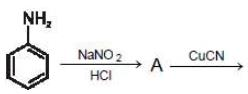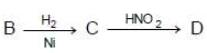Solution:
QUESTION: 98

In a reaction of aniline a coloured products C was obtained. The structure of C would be :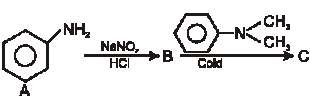Solution:
QUESTION: 99

In the reaction the compound (A) is known as :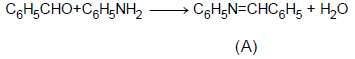Solution:
QUESTION: 100

Consider the following reaction What is Y?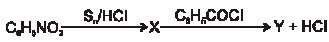Solution:
QUESTION: 101

The following questions consist of two statements one  labelled ASSERTION (A) and the another labelled REASON (R). Select the correct answers to these questions from the codes given below :

Assertion : Half-life period of a reaction of first order is independent of initial concentration.
Reason : Half-life period for a first order reaction t1/2 =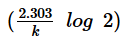Solution:
QUESTION: 102

The following questions consist of two statements one  labelled ASSERTION (A) and the another labelled REASON (R). Select the correct answers to these questions from the codes given below :

Assertion : If the activationenergyof a reactionis zero, temperature will have no effecton the rate constant.
Reason : Lower the activation energy, faster is the reaction

Solution:
QUESTION: 103

The following questions consist of two statements one labelled ASSERTION (A) and the another labelled REASON (R). Select the correct answers to these questions from the codes given below :

Assertion : Sodium ions are discharged in preference to hydrogen ions at a mercury cathode.
Reason : The nature of the cathode can effect the order of discharge of ions.

Solution:
QUESTION: 104

The following questions consist of two statements one labelled ASSERTION (A) and the another labelled REASON (R). Select the correct answers to these questions from the codes given below :

Assertion : The standard electrode potential of a half cell has a fixed value.
Reason : A negative value of standard reduction potential means that reduction take place on this electrode with reference to standard hydrogen electrode

Solution:
QUESTION: 105

The following questions consist of two statements one labelled ASSERTION (A) and the another
labelled REASON (R). Select the correct answers to these questions from the codes given below :

Assertion : Molecules of adsorbate and adsorbent are held by van derWaal's forces in physisorption and by chemical bonds in chemisorption.
Reason : The enthalpy of physisorption is greater than chemisorption

Solution:
QUESTION: 106

The following questions consist of two statements one labelled ASSERTION (A) and the another labelled REASON (R). Select the correct answers to these questions from the codes given below :

Assertion : An emulsion becomes stable if soap is added to it .
Reason : Soap contains hydrophilic and hydrophobic parts.

Solution:
QUESTION: 107

The following questions consist of two statements one labelled ASSERTION (A) and the another labelled REASON (R). Select the correct answers to these questions from the codes given below

Assertion : Leaching involves treatment of the ore with a suitable reagent so as to make it soluble while impurities remains insoluble.
Reason : Leaching is a process of reduction.

Solution:
QUESTION: 108

The following questions consist of two statements one labelled ASSERTION (A) and the another labelled REASON (R). Select the correct answers to these questions from the codes given below :

Assertion : In Halland Heroult's process,Al is extracted by electrolysis of a fusedmixture of alumina,cryolite and fluorspar.
Reason : Addition of cryolite and fluorspar lowers the fusion temperature and increases the conductivity of the electrolyte

Solution:
QUESTION: 109

The following questions consist of two statements one labelled ASSERTION (A) and the another labelled REASON (R). Select the correct answers to these questions from the codes given below :

Assertion : All the octahedral complexes of Ni2+ must be outer orbital complexes.
Reason : Outer orbital octahedral complexes are given weak ligands

Solution:
QUESTION: 110

The following questions consist of two statements one labelled ASSERTION (A) and the another labelled REASON (R). Select the correct answers to these questions from the codes given below :

Assertion : Potassiumferrocyanide is diamagnetic whereas potassiumfericyanide is paramagnetic.
Reason : Crystal field splitting in ferrocyanide ion is greater than that of ferricyanide ion.

Solution:
QUESTION: 111

The following questions consist of two statements one labelled ASSERTION (A) and the another labelled REASON (R). Select the correct answers to these questions from the codes given below :

Assertion :At room temperature, oxygen exists as a diatomic gas, whereas sulphur exists as solid.
Reason : The catenated –O–O–O– chain are less stable as compared to O=O molecule.

Solution:
QUESTION: 112

The following questions consist of two statements one labelled ASSERTION (A) and the another labelled REASON (R). Select the correct answers to these questions from the codes given below :

Assertion : Reaction of SO2 and H2S in the presence of Fe2O3 catalyst gives elemental sulphur.
Reason : SO2 is a reducing agent.

Solution:
QUESTION: 113

The following questions consist of two statements one labelled ASSERTION (A) and the another labelled REASON (R). Select the correct answers to these questions from the codes given below :

Assertion : AgCl dissolves in NH4OH solution.
Reason : Due to formation of a complex

Solution:
QUESTION: 114

The following questions consist of two statements one labelled ASSERTION (A) and the another
labelled REASON (R). Select the correct answers to these questions from the codes given below :

Assertion : Nitration of toluene is slow than benzene.
Reason : The methyl group in toluene is electron-withdrawing.

Solution:
QUESTION: 115

The following questions consist of two statements one labelled ASSERTION (A) and the another labelled REASON (R). Select the correct answers to these questions from the codes given below :

Assertion : Halogens are deactivating groups but have ortho-para directing nature.
Reason : Deactivating nature of halogen can be explain by –I & ortho-para directing nature can be explain by stability of intermediate by + M.

Solution:
QUESTION: 116

The following questions consist of two statements one labelled ASSERTION (A) and the another labelled REASON (R). Select the correct answers to these questions from the codes given below :

Assertion : Nitrobenzene, gives metanitrotoluene on reaction with CH3Cl/AlCl3.
Reason : Nitro group is orthro,para directing

Solution:
QUESTION: 117

The following questions consist of two statements one labelled ASSERTION (A) and the another labelled REASON (R). Select the correct answers to these questions from the codes given below :

Assertion : Diphenyl can not be prepared by following reaciton .Reason : Phenyl chloride is veryweakly ionised by AlCl3 because Ph+ carbocation is very less stable

Solution:
QUESTION: 118

The following questions consist of two statements one labelled ASSERTION (A) and the another labelled REASON (R). Select the correct answers to these questions from the codes given below

Assertion : 1-Hydroxypropanone is oxidised by HIO4
Reason : Glycol on HIO4 oxidation gives formic acid.

Solution:
QUESTION: 119

The following questions consist of two statements one labelled ASSERTION (A) and the another labelled REASON (R). Select the correct answers to these questions from the codes given below :

Assertion : Iodide (I-) is the strongest nucleophile (in protic solvent) among the halide anions
Reason : Iodide is the largest halide anion and is the most weakly solvated in a protic solvent

Solution:
QUESTION: 120

The following questions consist of two statements one labelled ASSERTION (A) and the another labelled REASON (R). Select the correct answers to these questions from the codes given below :

Assertion :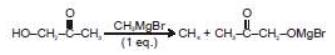Reason : In above reaction Grignard reagent react with hydroxyl group due to active hydrogen.

Solution:
QUESTION: 121

Which one of the following generally acts as an antagonist to gibberellins ?

Solution:
QUESTION: 122

The rate of growth of any organism follows

Solution:
QUESTION: 123

Osmotic pressure is maximum in

Solution:
QUESTION: 124

Amount by which water potential is reduced due to presence of solute is called

Solution:
QUESTION: 125

Substances that have a ________________moiety, find it difficult to pass through the membrane.

Solution:
QUESTION: 126

The movement of materials through the vascular tissue of plants is called

Solution:
QUESTION: 127

Which of the following statement does not hold true for restriction enzyme?

Solution:
QUESTION: 128

Arithmetic growth can be mathematically expressed as

Solution:
QUESTION: 129

The chemical nature of kinetin is

Solution:
QUESTION: 130

Transport methods those require special memberne proteins also show :

Solution:
QUESTION: 131

The simplest expression of arithmetic growth is examplified by

Solution:
QUESTION: 132

Match the following (Wrt. Discovery of PGRS)
(a) Canary grass                (i) Ethylene
(b) Bakane of rice              (ii)Auxin
(c) Tobacco stem callus     (iii)GA
(d) Ripened orage              (iv) Cytokinins

Solution:
QUESTION: 133

Which of the following is ultimately responsible for enlargement and extension growth of cells ?

Solution:
QUESTION: 134

Guttation is caused due to

Solution:
QUESTION: 135

Read the following four statements (A-D) about certain mistakes in two of them
(A) Retroviruses are not used as a vector in making transgenic animals by r-DNA technology
(B) Restriction enzymes are used in isolation of DNA from other macro-molecules
(C) Downstream processing is one of the steps of r-DNA technology
(D) Disarmed pathogen vectors are also used in transfer of r-DNA into the host
Which are the two statements having mistakes ?

Solution:
QUESTION: 136

Due to low atmospheric pressure, the rate of transpiration will

Solution:
QUESTION: 137

Which of the following one provide protection to the DNA of bacteria from its restriction endonuclease.

Solution:
QUESTION: 138

In genetic engineering, a DNA segment (gene) of interest, is transferred to the host cell through a vector. Consider the following four agents (A-D) in this regard and select the correct option about which one or more of these can be used as a vector/vectors:
(A) a bacterium (B) plasmid (C) plasmodium (D) bacteriophage

Solution:
QUESTION: 139

How many DNA molecules can be formed by a DNA molecule after twelve cycles of PCR

Solution:
QUESTION: 140

In transgenics, the expression of transgene in the target tissue is known by

Solution:
QUESTION: 141

Identify the true statement/s
(a) Hepatitis-B is a chronic infection
(c) excessive doses of coccaine cause hallucinations
(d) An antitoxin is generally a preparation containing antibodies to toxin

Solution:
QUESTION: 142

Identify the true statement/s
(a) Inbreeding is necessary if we want to evolve pureline in any animal
(b) outcross is the best breedingmethod for animals t at are belowaverage in productivity inmilkproduction
(c) Hilsa is most common edible fresh water fish commonly used in coastal india
(d) Hirsadale is a sheep developed in punjab by using technique of interspecific hybridisation

Solution:
QUESTION: 143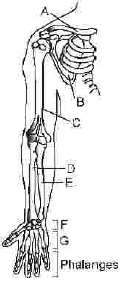The accompanied diagramshows right pectoral girdle and upper arm (frontal view). Identify A to G

Solution:
QUESTION: 144

Following is the figure of actin (thin) filaments. Identify A, Band C.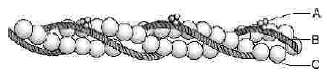Solution:
QUESTION: 145

Match the columns -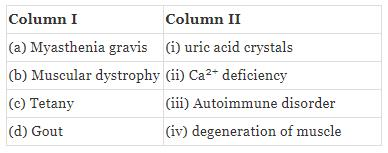Solution:
QUESTION: 146

Choose the letter from the figure that most appropriately corresponds to the structure -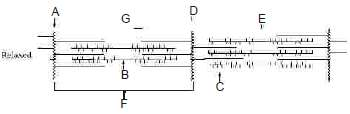I.A-band
II. I-band
III. Sarcomere
IV. H-zone
V.Myosin
VI. Actin, Troponin, Tropomyosin
VII. Z-line

Solution:
QUESTION: 147

Match the column
(1) examination of                 (a) PET scan multiple sclerosis
(2) Detection of spina bifida  (b) Endoscopy
(3) Tumour of stomach         (c) MRI
(4) Study of schizophrenia    (d) sonography

Solution:
QUESTION: 148

Match Column I with Column II -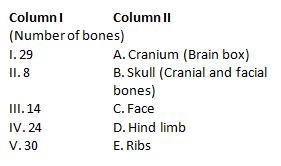Solution:
QUESTION: 149

Which one of the following statements is true regarding digestion and absorption of food in humans?

Solution:
QUESTION: 150

Which one of the following is a fat-soluble vitamin and its related deficiency disease?

Solution:
QUESTION: 151

Which one of the following is the correct matching of the site of action on the given substrate, the enzyme acting upon it and the end product?

Solution:
QUESTION: 152

Which of the following are required in less amount by human

Solution:
QUESTION: 153

Match the column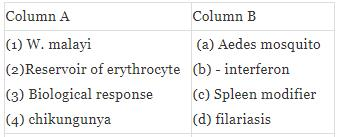Solution:
QUESTION: 154

Match the item in Column (vitamins) with those in Column (deficiency diseases)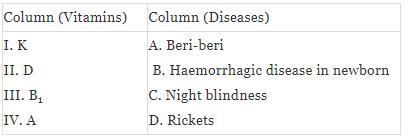Which one of the following is the correct matching of all the four vitamins

Solution:
QUESTION: 155

Probiotics are -

Solution:
QUESTION: 156

When breast feeding is replaced by less nutritive food low in proteins and calories; the infants below the age of one year are likely to suffer from

Solution:
QUESTION: 157

Given below are four statements (a-d) regarding human blood circulatory system
(a) Arteries are thick-walled and have narrow lumen as compared to veins
(b) Angina is acute chest pain when the blood circulation of the brain is reduced
(c) Persons with blood group AB can donate blood to an person with any blood group under ABOsystem
(d) Calcium ions play a very important role in blood clotting
Which two of the above statements are correct

Solution:
QUESTION: 158

Which one of the following statements is correct regarding blood pressure

Solution:
QUESTION: 159

Match the column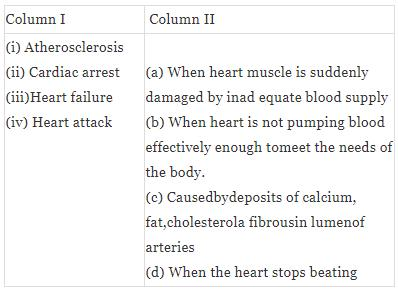Solution:
QUESTION: 160

Match the column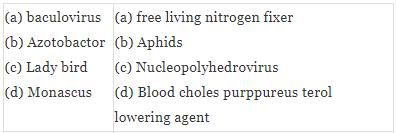Solution:
QUESTION: 161

The following questions consist of two statements one labelled ASSERTION (a) and the another labelled REASON (R). Select the correct answers to these questions from the codes given below :

Assertion : Winter varieties of wheat & rye are planted in autumn not in spring.
Reason : Winter varieties planted in spring. Normally they fail to flower or produce mature grain within a span of flowering season.

Solution:
QUESTION: 162

The following questions consist of two statements one labelled ASSERTION (a) and the another labelled REASON (R). Select the correct answers to these questions from the codes given below :

Assertion : Secondary xylem and secondary phloem both are redifferentiated tissue.
Reason : Both of the tissue once again lose the capacity to divide, but mature to perform specific functions.

Solution:
QUESTION: 163

The following questions consist of two statements one labelled ASSERTION (a) and the another labelled REASON (R). Select the correct answers to these questions from the codes given below :

Assertion : Buttercup produces two different kind of leaves within the same plant body.
Reason : Plants follow different pathways in response to environment or phases of life to form different kinds of structures, due to plasticity.

Solution:
QUESTION: 164

The following questions consist of two statements one labelled ASSERTION (a) and the another labelled REASON (R). Select the correct answers to these questions from the codes given below :

Assertion : Gibberellin application increase sugarcane yield as much as 20 tonnes per acre.
Reason : Gibberellin increases the length of the stem of the sugarcane

Solution:
QUESTION: 165

The following questions consist of two statements one labelled ASSERTION (a) and the another labelled REASON (R). Select the correct answers to these questions from the codes given below :

Asseration : Water is often the limiting factor for plants growth and productivity in both agriculatural and natural environment.
Reason : Plants show high demand of water for growth development

Solution:
QUESTION: 166

The following questions consist of two statements one labelled ASSERTION (a) and the another labelled REASON (R). Select the correct answers to these questions from the codes given below :

Asseration : The techniques of genetic engineering hav great advantage over traditional hybridisation procedures used in plant and animal breeding.
Reason : Recombinant DNA techology allows us to isolated and introduce only desirable gens with out introducing undesirable genes into the target organism.

Solution:
QUESTION: 167

The following questions consist of two statements one labelled ASSERTION (a) and the another labelled REASON (R). Select the correct answers to these questions from the codes given below :

Asseration : Most of the water flow in roots occurs via the apoplastic path.
Reason : Cortex largest region of root offer no resitance to water due to intercellur space

Solution:
QUESTION: 168

The following questions consist of two statements one labelled ASSERTION (a) and the another labelled REASON (R). Select the correct answers to these questions from the codes given below :

Asseration : Loss of solute from phloem during unloding produces low water potential in phloem.
Reason : Low water potential of phloem after unloading is responsible for returning of water to xylem.

Solution:
QUESTION: 169

The following questions consist of two statements one labelled ASSERTION (a) and the another labelled REASON (R). Select the correct answers to these questions from the codes given below :

Assertion:In day neutral plants there is no co-relation between exposure to light duration and flowering response.
Reason : In day neutral plant there is no specific requirement of light and dark hours.

Solution:
QUESTION: 170

The following questions consist of two statements one labelled ASSERTION (a) and the another labelled REASON (R). Select the correct answers to these questions from the codes given below :

Asseration : Green revolution succeeded in tripling the food supply.
Reason : It is mainly due to the use of better management practices and use of agrochemicals

Solution:
QUESTION: 171

The following questions consist of two statements one labelled ASSERTION (a) and the another labelled REASON (R). Select the correct answers to these questions from the codes given below :

Assertion : Yeast such as saccharomyces cerevisiae are used in baking industry.
Reason : Carbon dioxide produced during fermentation causes bread dough to rise by thermal expansion.